# What is the key points in Laser Optics Propagation

In many laser optics propagation applications, the laser optics beam is assumed Gaussian shape with an irradiance profile that follows an ideal Gaussian. All actual laser optics will have some deviation from ideal Gaussian behavior. What is the key parameters in laser optics propagation? There articles will share a bit about it.

#### 3 Key parameters

In most of our design works, we uses terms such as Waist and Angle to describe a Gaussian beam. Beam Size, Divergence and Rayleigh Range theta are used to report the beam characteristics as it propagates from surface to surface.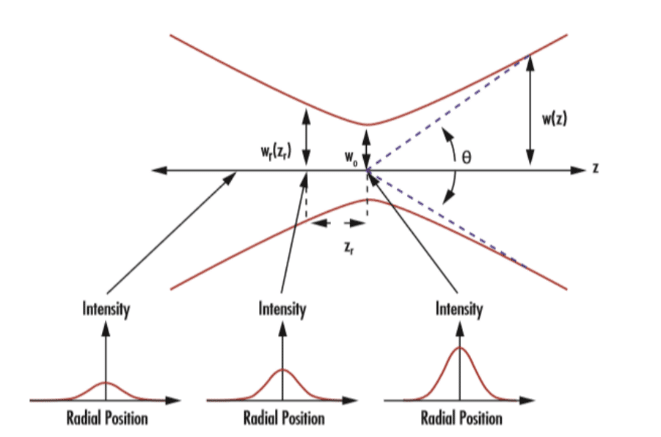The Gaussian waist is where the beam size reaches its minimum. Waist w0 is the half width or radius of the laser optics at its thinnest. We use the half divergence angle represented by theta/2 to describe the beam divergence.

The beam size is a function of the distance from the waist, z: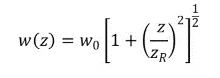The half divergence angle of the beam is given by: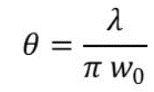Here, zis the Rayleigh range of the beam given by: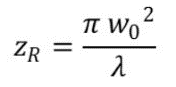The phase radius of curvature of the beam is a function of the distance from the beam waist, z: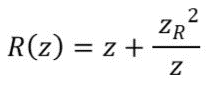The wavefront of the laser optics is planar at the beam waist and approaches that shape again as the distance from the beam waist region increases.

Many laser optics systems require manipulation of a laser beam as opposed to simply using the “raw” beam. This may be done using optical components such as lenses, mirrors, prisms, etc.

The behavior of an ideal thin lens can be described using the following equation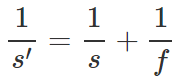#### Laser Optics Focal Shift

This phenomenon is  called Gaussian focal shift in laser beam focusing, it says that the intensity of a focused beam in a target at a fixed distance (L) away from the lens is not maximized when the waist is located at the target. The intensity on the target is actually maximized when the waist occurs at a location before the target.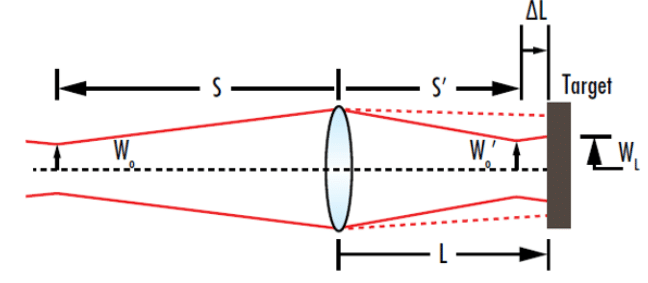#### Collimating a Laser optics Beam

Achieving a truly collimated beam where the divergence is 0 is not possible, but achieving an approximately collimated beam by either minimizing the divergence or maximizing the distance between the point of observation and the nearest beam waist is possible. To collimate a Laser optics beam, the distance from the beam waist to the collimating lens should be equal to the lens’ focal length.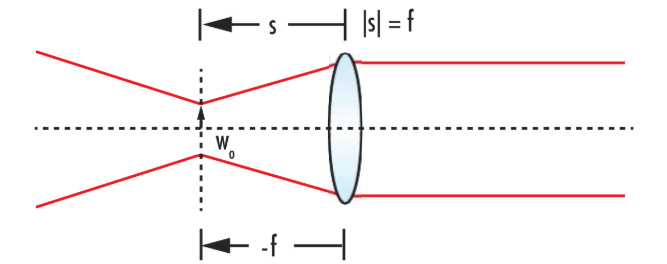#### Focusing a Laser optics Beam to a Spot

In many laser optics applications, such as materials processing or surgery, focusing a laser beam down to the smallest possible size is crucial for a wide range of applications. In this example as below, a magnification of 2, the output beam waist will be twice the input beam waist and the output divergence will be half of the input beam divergence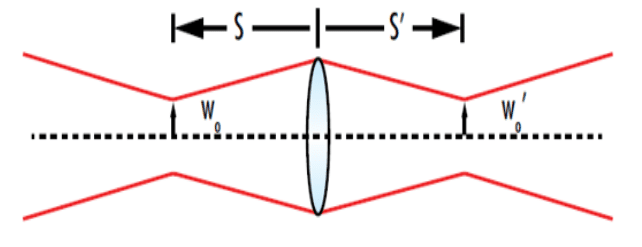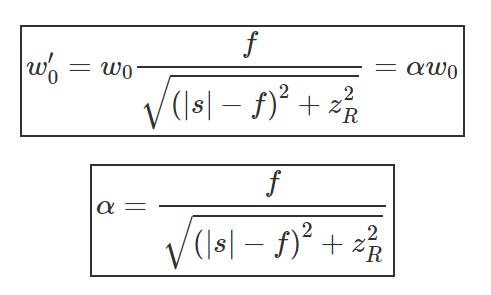From the equation above, we can see that the focused beam waist can be minimized by reducing the focal length of the lens and |s|-f.

Reference  Source:

https://www.zemax.com/

Zemax Optical Design Program User’s Guide, Zemax Development Corporation

https://www.edmundoptics.com/knowledge-center/application-notes/lasers/gaussian-beam-propagation/

https://en.wikipedia.org/wiki/Main_Page

### Disclaimer:

The material used in this knowledge sharing, is only for research, academic, non-profit educational or personal use, the blog owner has strived to credit the original sources, but cannot warrant the accuracy of copyrights or completeness of the information sources.